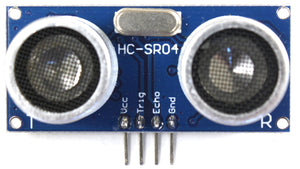Ultrasonic Distance Sensor and WeMos Tutorial

This tutorial will help you start receiving data from your HC SR04 Distance Sensor. This will allow you to add a distance sensor to your projects and be able to tell how far objects are away from it.

How Does the Ultrasonic Sensor Work

The sensor emits a 40kHz 8 cycle ultrasonic sound burst from the transmitting end. The burst then hits and bounces off objects in front of it returning the Ultrasonic 8 cycle burst to the receiving end. Because the speed of sound is a constant you can calculate the distance from the sensor to the object hit by its ultrasonic sound. By dividing the travel time of the ultrasonic burst by 58 you get the distance measured in centimeters. If you divide by 148 instead you get the distance measured in inches.Connecting the Ultrasonic Distance Sensor to WeMos

The following image should help you in connecting the WeMos to the Distance Sensor.Connection Map

 HC-SR04 Distance Sensor WeMos D1 Mini Vcc 5V Trig D5 Echo D6 Gnd G

Code for the WeMos D1 Mini

Upload the following code to your WeMos D1 Mini and the hookup the distance sensor as shown above and you should start getting feedback from the serial monitor.

``````// defines pins numbers
const int trigPin = D5;
const int echoPin = D6;
// defines variables
long duration;
int distance_cm;
int distance_in;
void setup() {
pinMode(trigPin, OUTPUT); // Sets the trigPin as an Output
pinMode(echoPin, INPUT); // Sets the echoPin as an Input
Serial.begin(9600); // Starts the serial communication
}
void loop() {
// Clears the trigPin
digitalWrite(trigPin, LOW);
delayMicroseconds(2);
// Sets the trigPin on HIGH state for 10 micro seconds
digitalWrite(trigPin, HIGH);
delayMicroseconds(10);
digitalWrite(trigPin, LOW);
/* Reads the echoPin, returns the sound wave
travel time in microseconds */
duration = pulseIn(echoPin, HIGH);
// Calculating the distance
distance_cm = duration / 58;
distance_in = duration / 148;
/* Prints the distance on the Serial Monitor
Serial.print("Distance: ");
Serial.print(distance_in);
Serial.println(" inches"); */
Serial.print("Distance: ");
Serial.print(distance_cm);
Serial.println(" centimeters");
/* uncomment the 3 Serial.print lines
to display measure in inches */
}
``````

The above code will print the measurement results to the serial monitor. To change to inches instead of centimeters uncomment the three lines labeled Serial.print and comment three lines following so you can read the output better.

Comment below and let us know if this code has helped you with your project.